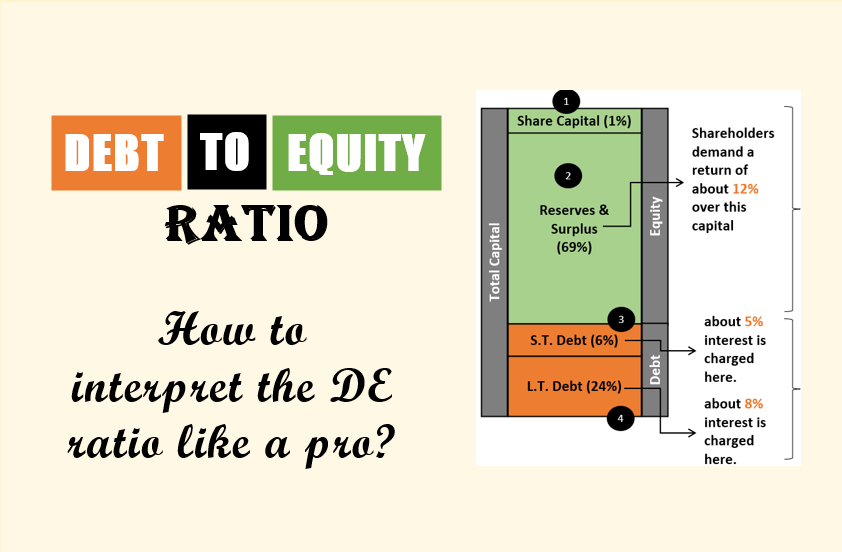# Category: Stock BasicsStock Basics

### Debt To Equity Ratio – Basics, Formula, Calculations, and Interpretation

The debt-Equity ratio (DE) is easy to calculate. But its interpretation is not as straightforward as we think. We generally assume that a DE ratio of 2 is acceptable. But for analysts, this number is too high. Let’s look at the DE ratio from an analyst’s perspective.